# The Inspector Program

The Inspector program allows you to see the actual lvalue and rvalue of a variable. Figure 4.3 shows a sample run of the program.

##### Figure 4.3. The Inspector program.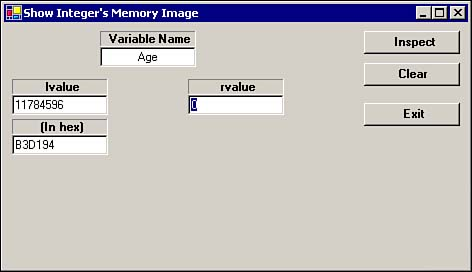As you can see, the program has a defined variable named Age , and it has asked the Windows operating system for enough memory (that is, 4 bytes) to store the Integer variable named Age . Evidently, the request is successful, as you can see from the lvalue of 11784596 . (The program displays the memory address in hexadecimal notation as well as in decimal because that is the numbering system that Windows uses for memory addresses.) You can also see from Figure 4.3 that the Age variable has been initialized with the value of , which is the current (default) rvalue for Age .

Now, type the number 34 into the rvalue text box and then click the Inspect button. Doing this produces the information shown in Figure 4.4.

##### Figure 4.4. Output of the Inspector program after the assignment statement is processed .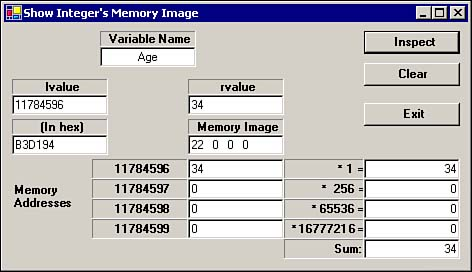Note the changes in the display. First, look at the text box below the Memory Image label. It shows these values:

` 22 0 0 0 `

This is the value 34 expressed in hexadecimal. (Because hexadecimal is a base-16 numbering system, you take the first 2, multiply it by 16 to get 32, and then add the second 2 to get 34.) Therefore, the first memory byte used by Age ”memory address 11784596 ”has the numeric value 34 stored in it. The other three memory addresses used by Age are all because the value 34 can be stored in a single byte. Therefore, any value stored in the first byte is scaled by the value 1. If you had a value stored in the second byte, it would be scaled by 256 because the first byte can only store a value between 0 and 255. Remember that

2 8 = 256

Because the first byte in an Integer data type uses 8 bits, anything stored in the second byte must be scaled by 256 to determine its actual (decimal) numeric value.

In similar fashion, anything stored in the third byte is scaled by this:

2 16 = 65536

and the fourth byte is scaled by this:

2 24 = 16777216

Adding all the values together determines the final Integer value that is stored in the 4 bytes associated with the variable named Age .

Try using a value for Age that is larger than will fit in a single byte: Use an rvalue of 1000 . The result is shown in Figure 4.5.

##### Figure 4.5. The Inspector program, using the rvalue 1000 .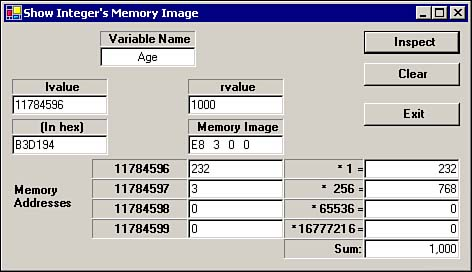When you do the conversion, the hex value E8 , as stored in the first byte, is 232 in decimal. When multiplied by its scalar value of 1, its value is 232 . The second byte of the Integer variable Age has the value 3 . After it is multiplied by its scalar value of 256, its value becomes 768 (that is, 256 x 3 = 768). The remaining bytes for Age are , yielding scaled values of . Adding the numbers produces a decimal value of 1,000, which is exactly what the rvalue of Age should be.

Figure 4.6 shows one more run of the Inspector program, using the one million for the rvalue .

##### Figure 4.6. The Inspector program, using the value of 1,000,000.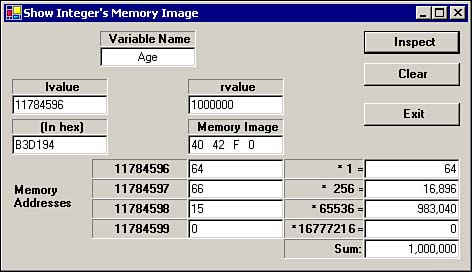You should be able to convince yourself that the proper rvalue has been assigned to Age .

There is one more thing to notice in Figures 4.3 through 4.6. In each run of the program, the lvalue of Age does not change. Although this has no real impact on how the program runs, it does illustrate an important point: It is the operating system, not Visual Basic .NET, that determines the lvalue for a variable. For this reason, a Visual Basic .NET program you write should never attempt to change the lvalue of a variable. If you tried to change an lvalue , you might assign a value into a piece of memory that Windows is using for some other purpose, and that is almost never a good thing!

One more thing you can prove by using the Inspector program is that Windows stores memory addresses in a rather weird way. If you type in the lvalue (that is, 11784596 ) as the rvalue , you should see the lvalue expressed in hex in the Memory Image text box. This is shown in Figure 4.7.

##### Figure 4.7. The Inspector program, using the lvalue of Age as the rvalue .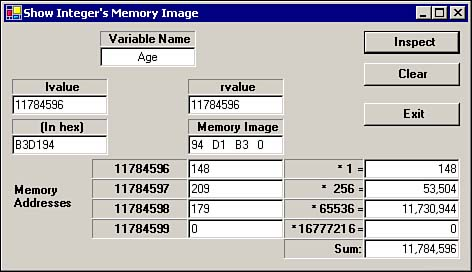Notice that the lvalue in hex is B3D194 , but the rvalue is stored as 94 D1 B3 . Yet when you look at the scaled number, everything works out correctly. The reason it works this way is because the CPU stores memory addresses with the least significant memory byte first, progressing to the most significant memory bytes. There are some technical reasons the chipmakers use this format for memory addresses, but you need not concern yourself about that at this point. Still, you might want to keep this tidbit of information in mind because you never know when this topic will come up at a cocktail party.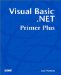Visual Basic .NET Primer Plus
ISBN: 0672324857
EAN: 2147483647
Year: 2003
Pages: 238
Authors: Jack Purdum

Similar book on Amazon